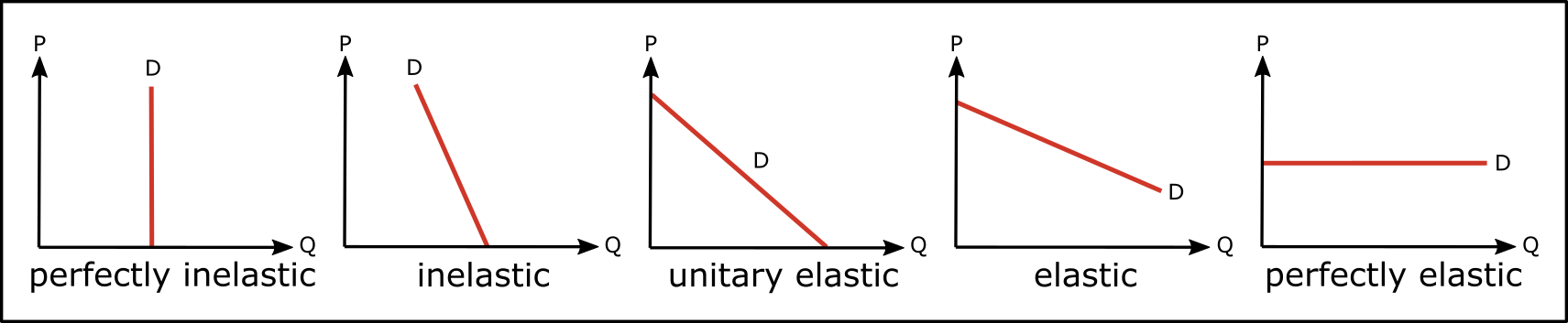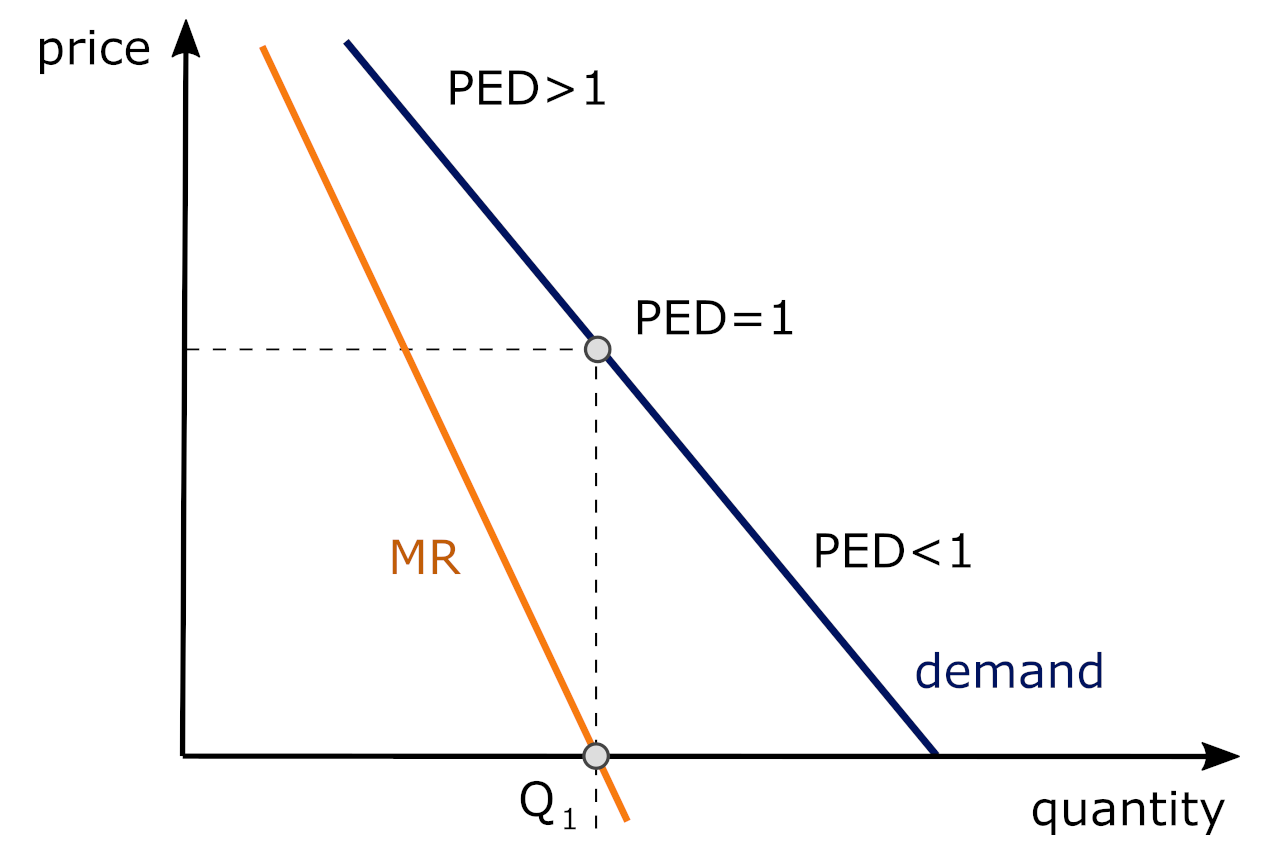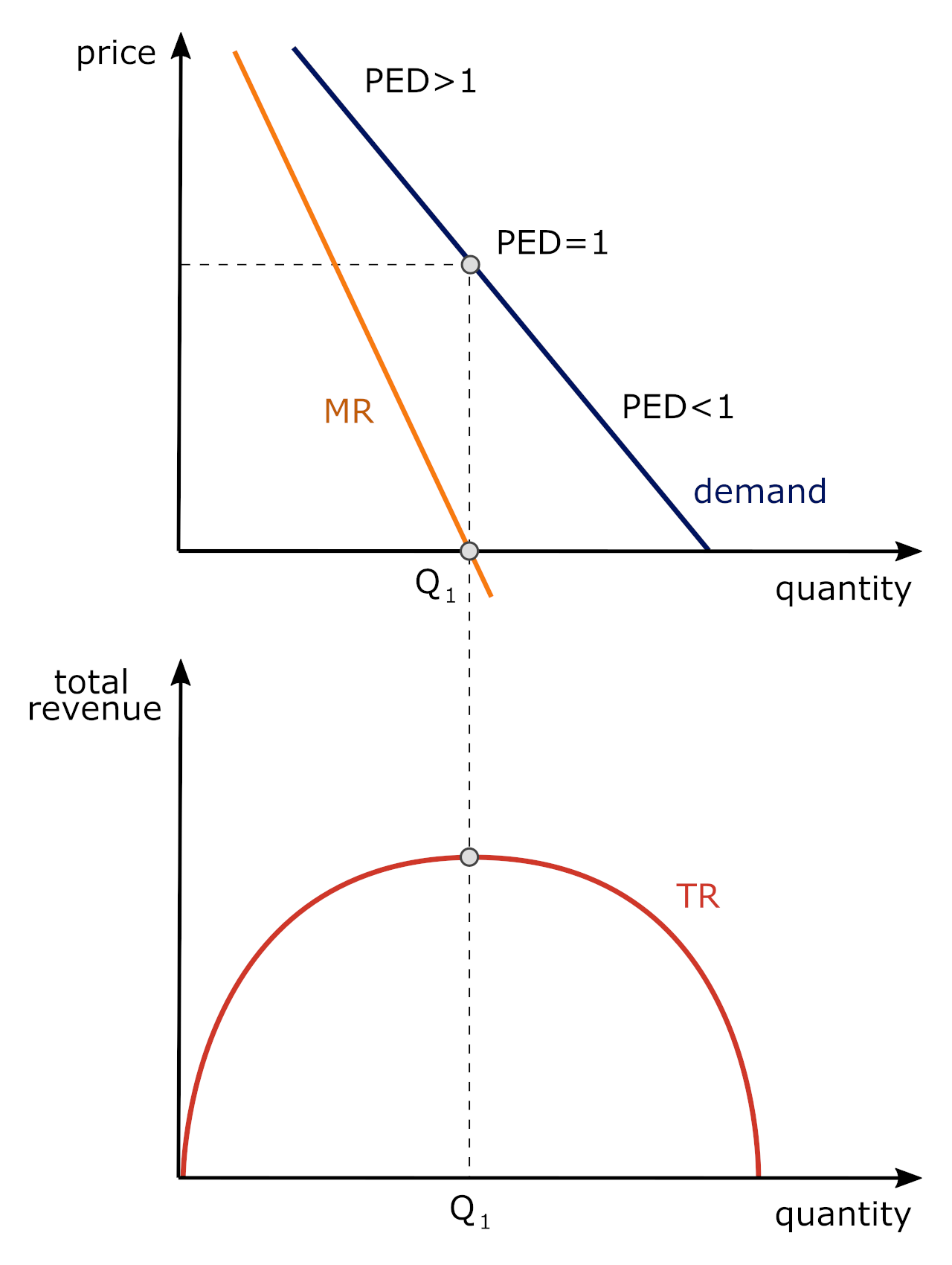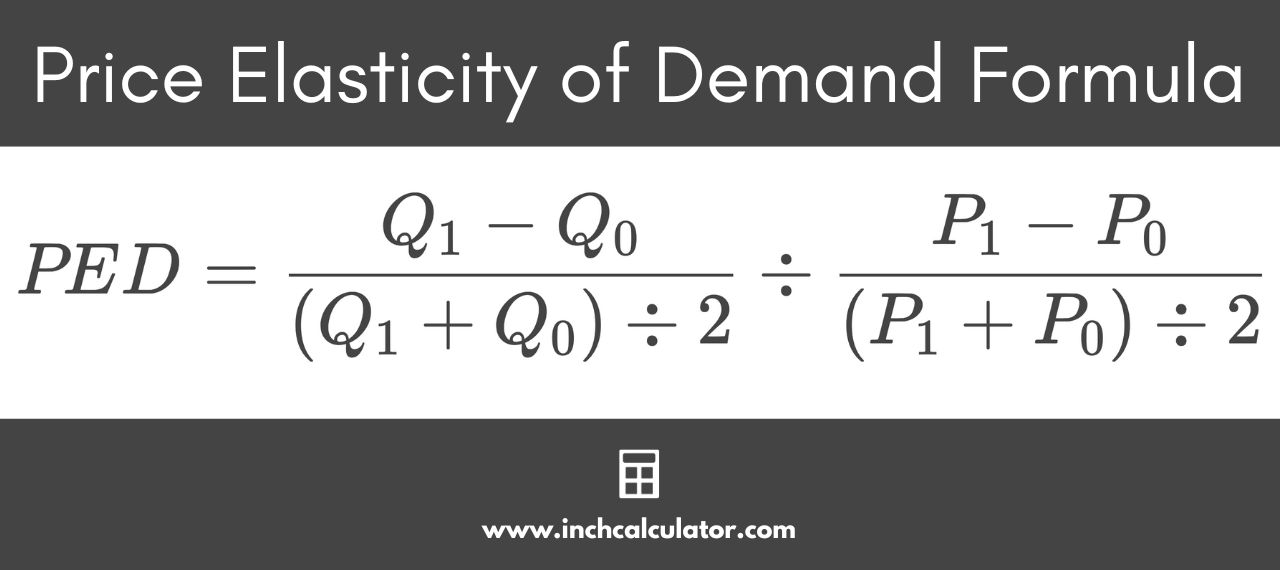# Price Elasticity of Demand Calculator

Use our price elasticity of demand calculator to calculate the change in the demand for goods and services.

Initial Price and Demand
$Final Price and Demand$

## Price Elasticity of Demand:

 PED: Elasticity:Initial Revenue: $Final Revenue:$ Revenue Growth: $Learn how we calculated this below ## On this page: ## How to Calculate Price Elasticity of Demand There is no denying that when the price of something changes, the quantity demanded for that product will also change. When the price of a good decreases, the number of people who are willing to buy that good will directly increase. At the same time, when the price of a good increases, fewer people will be willing and able to buy that good – even if that good is something that most people consider to be essential. This behavior can be easily observed with changing gas prices. When gas is relatively inexpensive, people will be more willing to drive and use their car as their primary form of transportation. On the other hand, when gas prices are higher, people will typically drive less or be more willing to explore other forms of transportation. In addition to the elasticity of demand for gas when the price changes, there is also a cross-price elasticity for the demand for other goods and services such as cars or mass transit; you can calculate that using our cross-price elasticity calculator. However, contrary to what some people might assume, the rate at which people’s behaviors change is rarely linear. While the slope of the demand curve could be constant and linear, the elasticity is not, even with a demand “curve” with a constant slope. The image below illustrates this nicely. As we move along the demand curve from higher prices to lower prices, the price elasticity of demand changes (this is denoted by the greek symbol Eta, or η). At higher prices, the PED > 1 (in absolute value) but as prices fall then it becomes more inelastic. At the quantity where Marginal Revenue (MR) = 0, the PED is unit elastic since it equals unity. Marginal revenue is the change in total revenue from a change in output (i.e., % change in Total Revenue ÷ % change in output (Q)).The price elasticity of demand for a good depends upon the nature of the good. For example, goods that are considered necessary, tend to be “inelastic” while other goods, such as luxury items, are considered “elastic”. Whether you are an economist, a business owner, or someone who is simply curious, learning how to measure the price elasticity of demand can be very beneficial. Understanding price elasticity is crucial to determining the amount of markup a business might add to a product or service, and thus impacts the amount of margin they might earn. In particular, depending upon the price elasticity of demand coefficient (η), which indicates that the product has an inelastic or elastic demand, so that a firm can either raise (in the case of the former) or lower price (in the case of the latter) to sell more and increase total revenue (TR) as displayed in the illustration below:When Q is less than Q1, the firm is operating in the elastic portion of the demand curve, the firm can lower price to increase total revenue. However, when the firm produces in the inelastic portion of the demand curve, a decrease in price causes total revenue to decline (where Q > Q1). Note: where MR = 0 (Q = Q1), total revenue is maximized. (MR is the slope of the TR curve). ### What is Price Elasticity of Demand? The price elasticity of demand is an economic concept that explains how any change in the price of a good or service will affect the quantity demanded for that given good or service. A good is considered elastic if price changes have a strong impact on quantity demanded and inelastic f price changes have a limited effect on quantity demanded. There are many different variables that can affect price elasticity. If the good or service in question is considered a luxury rather than a necessity, then it will likely be much more elastic. Water, for example, is considered a somewhat inelastic product. While an increase in the price of water might mildly influence people’s behavior (taking shorter showers, running the dishwasher less, etc.), water is still an absolute necessity that people will always be willing to buy. Going to the movie theater, on the other hand, is generally considered a luxury and, therefore, the rate at which people go to the movies will be much more likely to change along with changing prices. This means the price of a movie ticket, at least when compared to water, should be considered elastic. This concept of price elasticity of demand will be crucial for anyone who is responsible for setting prices, forecasting future inventory needs, and making other important decisions. Let’s take a closer look at how to calculate the price elasticity of demand, along with some of the related variables you will need to keep in mind. ### Price Elasticity of Demand Formula In order to calculate the price elasticity of demand, you will need to know four different variables. These include the initial price of the good or service in question, its final price, the initial quantity, and the final quantity. Once you have determined these four variables, you can then utilize the following formula: η = PED = \frac{Q_{1} − Q_{0}}{(Q_{1} + Q_{0}) \div 2} \div \frac{P_{1} − P_{0}}{(P_{1} + P_{0}) \div 2} where: P0 = initial price P1 = final price Q0 = initial quantity Q1 = final quantity This formula is referred to as the midpoint formula, and is the formula that the calculator above and examples on this page use.There is an alternate formula that can also be used to calculate the price elasticity of demand, which interestingly yields different results in some cases than the midpoint formula. η = PED = \frac{Q_{1} − Q_{0}}{Q_{0}} \div \frac{P_{1} − P_{0}}{P_{0}} In most cases, using a calculator like the one above will be the easiest way to find the final price elasticity of demand coefficient (η), which should be negative. Generally speaking, it also helps to have a frame of reference to compare the product to determine whether the product is elastic or inelastic. ### Example: Calculating Price Elasticity of Demand Now that you understand the formula needed to calculate the price elasticity of demand, let’s take a look at an example. Suppose that a company sells a product for$10 and, at this price point, is able to sell 100 units of this product per month. However, as a result of supply chain challenges, the cost of acquiring this particular product increases, and the company then decides to increase the price of the product to $12 per month. Following the price hike, the monthly sales decreased from 100 units to only 60 units per month. This is all of the information you need to calculate the elasticity of demand. You can now use the following variables: P0 (initial price) =$10
P1 (final price) = $12 Q0 (initial quantity) = 100 units Q1 (final quantity) = 60 units You can now use the price elasticity of demand formula: η = PED = \frac{60 − 100}{(60 + 100) \div 2} \div \frac{12 − 10}{(12 + 10) \div 2} η = PED = -2.75 In this particular example, the product would be considered elastic. That means that a small change in the price had a strong impact on the quantity demanded for the product – after all, the price of the product only increased by$2 while the quantity demanded for the product decreased by 40 units.

Generally, something is considered elastic when the PED is greater than 1 (in absolute value – PED is always negative) or less than -1. If it’s between -1 and 1, it would be considered inelastic.

In the example above, the revenue created from selling the product at the original price, $10, was$1,000 per month. After the price increase, due to the decrease in quantity demanded, the revenue generated per month dropped down to just \$720.

This means the price increase resulted in a decrease in revenue, which is always the case when the PED > 1 in absolute value.

## What Factors Affect Price Elasticity of Demand?

There are several different factors that can affect the price elasticity of demand. The most notable factor is necessity. Goods and services that are considered necessities tend to be much more likely to be inelastic than goods and services that are considered luxuries.

Additionally, the availability of substitutes will also affect elasticity. If a person can easily replace a purchase with a comparable alternative, they will be more likely to stop buying the item following a price increase (meaning the item is elastic).

In general, as time passes, goods tend to become more elastic. For example, in the case of gasoline, people may buy more fuel-efficient vehicles and/or choose different modes of transportation.

Other variables, such as changes in income level and the general state of the economy, will also play an important role. Changes in income will not affect the price elasticity of demand; there is a concept known as income elasticity, which is where the changes in income come into play.

## Price Elasticity of Supply vs. Price Elasticity of Demand

The price elasticity of supply is very similar to the price elasticity of demand. Comparably, the price elasticity of supply demonstrates how changes in price will directly affect the quantity supplied of a good or service.